# How to Calculate a Monthly Lease Liability Amortization Schedule in Excel

Lucas Russell | 2021-05-04

In this how-to guide, we’ll cover how to calculate a monthly lease liability amortization schedule. This method shown is one of many ways of how to calculate a lease amortization schedule. The key attributes of this lease liability monthly amortization schedule are:

• One of the most common schedules you’ll see companies apply
• Uses the NPV function in Excel
• The calculation is done monthly
• It’s quicker to calculate compared to a daily lease liability amortization schedule
• It’s not as accurate compared the daily schedule due to:
- The NPV function does not take into account the dates of lease payments
- Cannot handle when modification accounting mid-month
- The calculation incurs interest regardless if the payment timing is In Advance or In Arrears

If you want to know more about the NPV Excel function and different ways to calculate the present value of a lease, refer to How to Calculate the Present Value of Future Lease Payments

Suppose you're looking for a more accurate way to calculate your lease liability that can handle any lease modification. I'd recommend this article, How to Calculate a Daily Lease Liability Amortization Schedule in Excel. I recommend the daily schedule as it’s not that much more of a time investment than the monthly NPV lease liability calculation we're about to go through. With the additional time investment, you have the most accurate lease liability calculation that factors in the exact timing of lease payments and allows you to handle any potential lease modification.

But in saying that, if the lease you're accounting for is straightforward, with a fixed payment frequency of monthly, you pay in advance, it's a relatively short lease term, and you expect no modifications, this calculation methodology should be sufficient.

If you would like the Excel calculations displayed in this article, reach out to [email protected].

## Lease Inputs

The lease agreement we’re going to calculate is based on the following details:

• Commencement date: January 1, 2021
• Lease end date: December 31, 2021
• Discount rate: 7%
• Fixed payment amount: \$10,000
• Payment timing: In Arrears

With these inputs, we'll calculate the monthly lease liability amortization schedule.

## Step 1 - Create the columns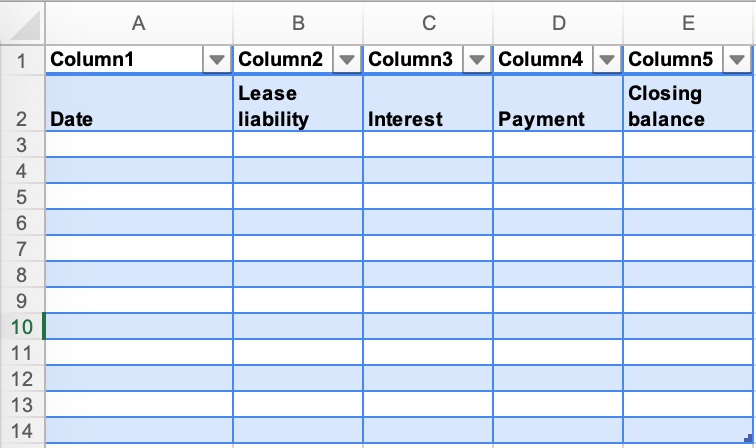• Create five columns within the Excel worksheet. Those columns will be called Date, Lease liability, Interest, Payment, Closing balance.

## Step 2 - Input the applicable dates and payments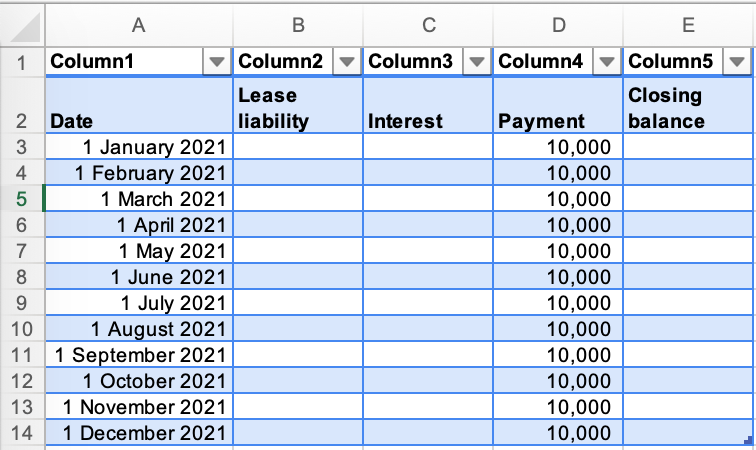• This data will be taken directly from the lease agreement. In this example, we have 12 payments, that occur on the last day of each month for an amount of \$10,000. We'll be working out the opening balance of the lease liability for each month.

## Step 3 - Apply the NPV function from Excel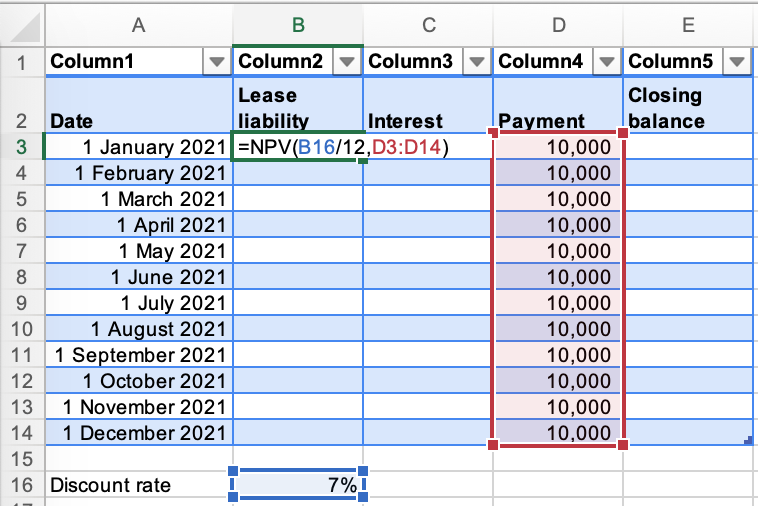• In the NPV formula, you must input the rate, which is the discount rate. You can see in the formula that the discount rate is divided by 12, given the monthly payments. This is a slight workaround to get a slightly more accurate NPV calculation.
• Here you can see the limitation of the NPV function as it does not consider the date of the payments.
• If the payments are in advance, you would not need to present value the first payment.

## Step 4 - Calculate the interest on the lease liability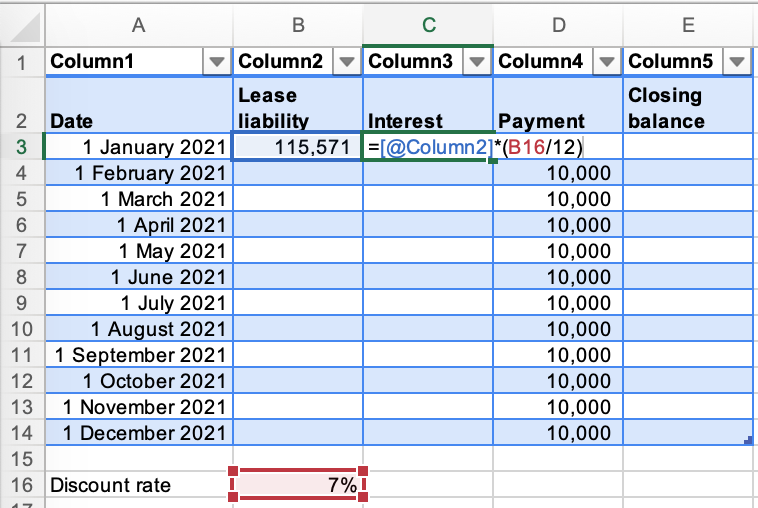• Select the lease liability amount and apply the applicable discount rate, this rate has been divided by 12 to be consistent with the NPV formula as the payments are monthly.

## Step 5 - Calculate the closing balance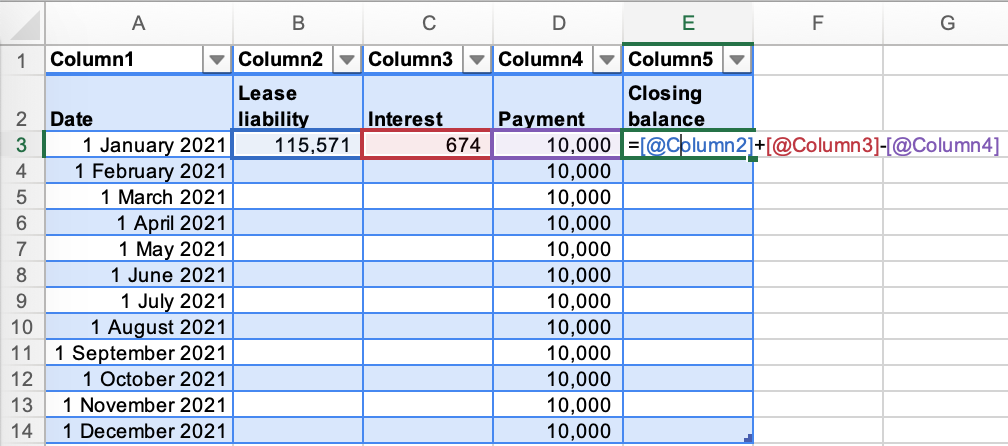• Calculate the closing balance of the lease liability at the end of the first month.

## Step 6 - Bring the closing balance forward for the next period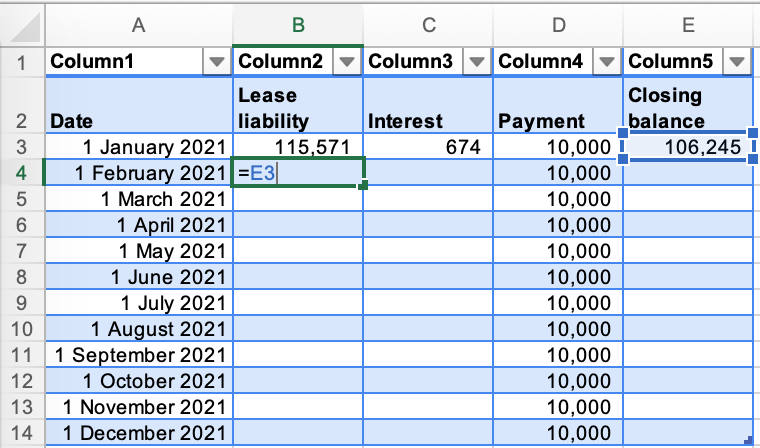• Apply the closing balance of the previous month, as that is opening balance for the next month.

## Step 7 - Input the formulas for each row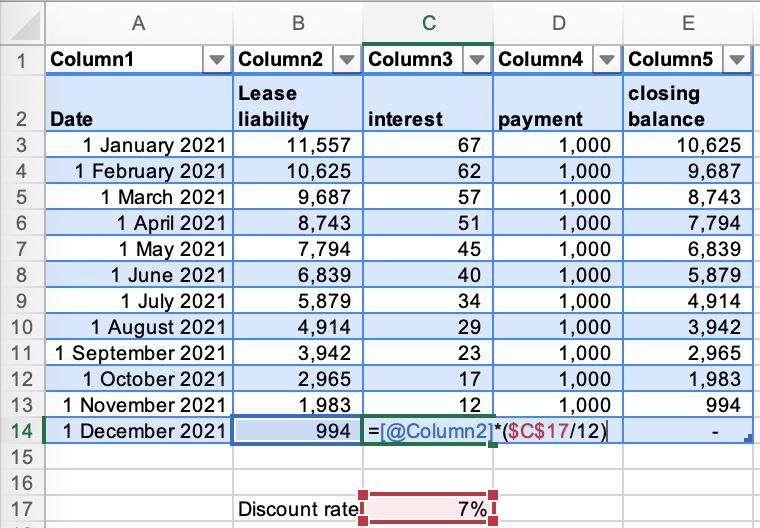• The closing balance of the lease liability should unwind to zero.
• Ensure your formulas are picking up the correct cells. If they are not, that's probably the reason why the lease liability amortization schedule is not unwinding to zero.
• Technically there should be no interest incurred in the month of December. The lease liability has been paid. This is a good example of the inaccuracy of calculating the lease liability on a monthly basis.

## Conclusion

We've gone through one way to calculate a lease amortization schedule. But that's just the tip of the iceberg when it comes to leasing accounting. I'd recommend the following articles which can add to your knowledge of lease accounting and save yourself a lot of time in the future:

## FooterHere at Cradle, our mission is simple; it's at the foundation of everything that we do. We want to make accountants' lives easier by leveraging technology to free up their time to focus on running the business.

140 Yonge St.
Suite 200
Toronto, ON M5C 1X6

US
Suite #73591
Torrance, CA 90503
USA

### Legal